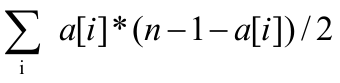Orzz(j)yf、kb都太强啦比我不知道高到哪里去了 Orz。。。

# T1 币 (coin)

$f[i][j]=1.0-(f[i-1][j]+f[i-1][j-1])/2$

#include <bits/stdc++.h>
using namespace std;
double f,ans;
int t,n;
int main()
{
freopen("coin.in","r",stdin),freopen("coin.out","w",stdout);
for(int i=1;i<=1000;i++)
for(int j=1;j<=i;j++)
f[i][j]=1.0-(f[i-1][j]+f[i-1][j-1])/2;
scanf("%d",&t);
while(t--)
{
scanf("%d",&n),ans=0;
for(int i=1,a;i<=n;i++)scanf("%d",&a),ans+=a*f[n][i];
printf("%.3lf\n",ans);
}
return 0;
}


# T2 △(triangle)#include <bits/stdc++.h>
using namespace std;
typedef long long LL;
static const LL maxs=100100;
queue<LL> que;
bool book[maxs+5];
int main()
{
freopen("triangle.in","r",stdin),freopen("triangle.out","w",stdout);
for(LL i=1,u,v;i<=m;i++)
{
scanf("%lld%lld",&u,&v);
if(u>v)swap(u,v);
push(u,v),ocnt[u]++,ocnt[v]++;
}
for(LL i=1;i<=n;i++)
{
tot+=ocnt[i]*(n-1-ocnt[i]);
while(!que.empty())
{
LL f=que.front();que.pop();
}
}
tot=n*(n-1)*(n-2)/6-tot/2;
printf("%lld %lld\n",ans,tot-ans);
return 0;
}


# T3 数 (aqnum)

1. 当 $i×p[j]$大于 upto，ans++，然后减掉。
2. 当 $j$大于 [1,maxprime] 中的质数个数时，ans++，然后减掉。
3. 当 $i×2×p[j]$大于 upto，但 $i×p[j]$小于等于 upto，直接二分找出满足条件的最大的 $j$设为 k，然后 $ans+=k-j+2$，然后减掉。

#include <bits/stdc++.h>
using namespace std;
typedef long long LL;
LL n,m,ans,p,psize;
bool book;
LL serch(LL k,LL l)
{
LL r=psize-1,mid;
while(mid=(l+r+1)>>1,l!=r)if(k*p[mid]<=n)l=mid;else r=mid-1;
return l;
}
void dfs(LL k,LL x)
{
if(x>=psize|k*p[x]>n){ans++;return;}
if(k*p[x]*p[x]>n){ans+=serch(k,x)-x+2;return;}
dfs(k,x+1),k*=p[x],dfs(k,x+1);
while(k*=p[x]*p[x],k<=n)dfs(k,x+1);
}
int main()
{
freopen("aqnum.in","r",stdin),freopen("aqnum.out","w",stdout);
scanf("%lld%lld",&n,&m);
for(LL i=2;i<=m;i++)
if(!book[i]){p[psize++]=i;for(LL j=(LL)i*i;j<=m;j+=i)book[j]=1;}
dfs(1,0),printf("%lld\n",ans);
return 0;
}